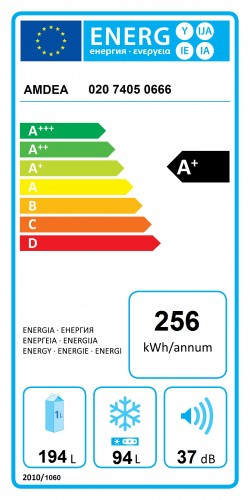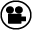# Intensity

Waves are all about the transmission of energy through a medium, whether that is mechanical, acoustical, electrical, etc. Let’s have a quick recap on energy:

Energy : unit = Joule. Cannot be created or destroyed – only turned from one form into another.

Power = energy flowing through a system in a given time: unit = Joule / s or WattEco labels on products such as fridges show sound power (37 dB in this case). This is a measure of the power being radiated by the fridge in all directions.

For waves, we often make use of another concept related to energy, Intensity. Intensity is the amount of wave power flowing through a square metre, perpendicular to the direction of wave travel.

Intensity = energy flowing through a certain area in a given time : unit = Joule / s / m2 or Watt / m2

If we can measure the power of a source of wave energy and we know over what area this power spreads out, we can work out the intensity of the wave at any point around that area. If the source is omnidirectional then the intensity may be the same where ever we look – but most sources direct more intensity in some directions than others, and are called directional sources.

Here’s another slow-motion video – a source of impulsive sound energy. If we were standing next to the light-bulb as it smashed, we’d expect it to be pretty loud…but if we were a long way away we might not hear it at all. Why is this?

# The inverse-square law

The inverse-square law is hugely important in physics. Without it, even gravity does not make sense. It can be pretty easy to understand using waves examples – but first let’s have a go using a silly, but useful, example of a party balloon.

Imagine taking a red balloon and blowing it up. What would you notice about the colour? The larger the balloon gets the paler the colour. Eventually it turns almost pink (or pops, but that’s not the point of our example).

You can think of the surface of the balloon as being like the wavefront radiating out from a wave source – say a loudspeaker. The depth of colour of the balloon is like the intensity of the wave. As the power (or total available amount of red rubber) has to spread out over a larger and larger area, it inevitably gets more thinly spread. The sound gets quieter (and the balloon looks paler). We’ve also heard this explained by thinking about the thickness of jam you could spread on a table -tennis ball, a cricket ball and a football, if you only have the same amount of jam (wave power) available in each case!

The key thing to remember is that the energy from the source is always the same – it is simply distributed over a larger area, the further away from the source you try to measure.

What has all this to do with the inverse-square law?

It comes down to the geometry of the area over which the power spreads out. Let’s make it easy for ourselves, and assume the power spreads out equally in all directions, in which case the area is the surface of a sphere. Therefore at a distance r from the source, the power P of the source passes through an area 4πr2 (the surface area of a sphere radius r).

I = P/A = P/ 4πr2

so I ∝ 1/r2 (∝ = proportional to)

This is the inverse square part. Square because the distance is squared, and inverse because intensity is proportional to one over distance squared.

For example: moving 10 metres away from a source will reduce wave intensity by a factor of 102 = 100.

The inverse-square law applies, where energy spreads out spherically.

Understanding the inverse square law is also vital to acoustical engineers

I was working on the environmental impact assessment of a new railway station, this included assessing the noise from taxis, trains, station announcements, etc. I needed to calculate how loud the noise would be at nearby homes, so I could work out if the noise was likely to cause problems. The inverse square law allowed me to work out how much quieter the noise got as it propagated through the air from the station to the homes.

Vicky Stewart, Acoustic ConsultantCareer case study: Why Vicky chose to study acoustical engineering

## Amplitude and intensity

The energy of a wave is proportional to the square of its amplitude. Therefore the intensity of a wave is also proportional to the square of its amplitude.

I ∝ A2

(∝ = proportional to)

This means that if the intensity drops off at a rate of 1/r2 , the wave amplitude drops off at a rate of 1/r. If we move twice as far from a loudspeaker, the sound intensity will decrease to one-quarter its original value, and the sound pressure amplitude will go down to one-half.

In acoustics, this is usually measured in decibels. When you travel twice as far from a loudspeaker, the sound pressure level drops by 6 decibels.